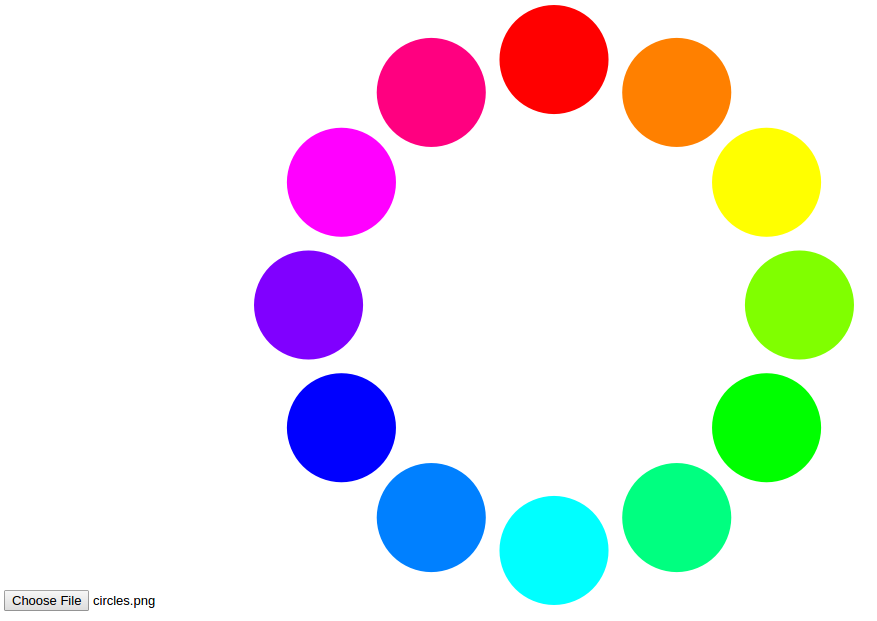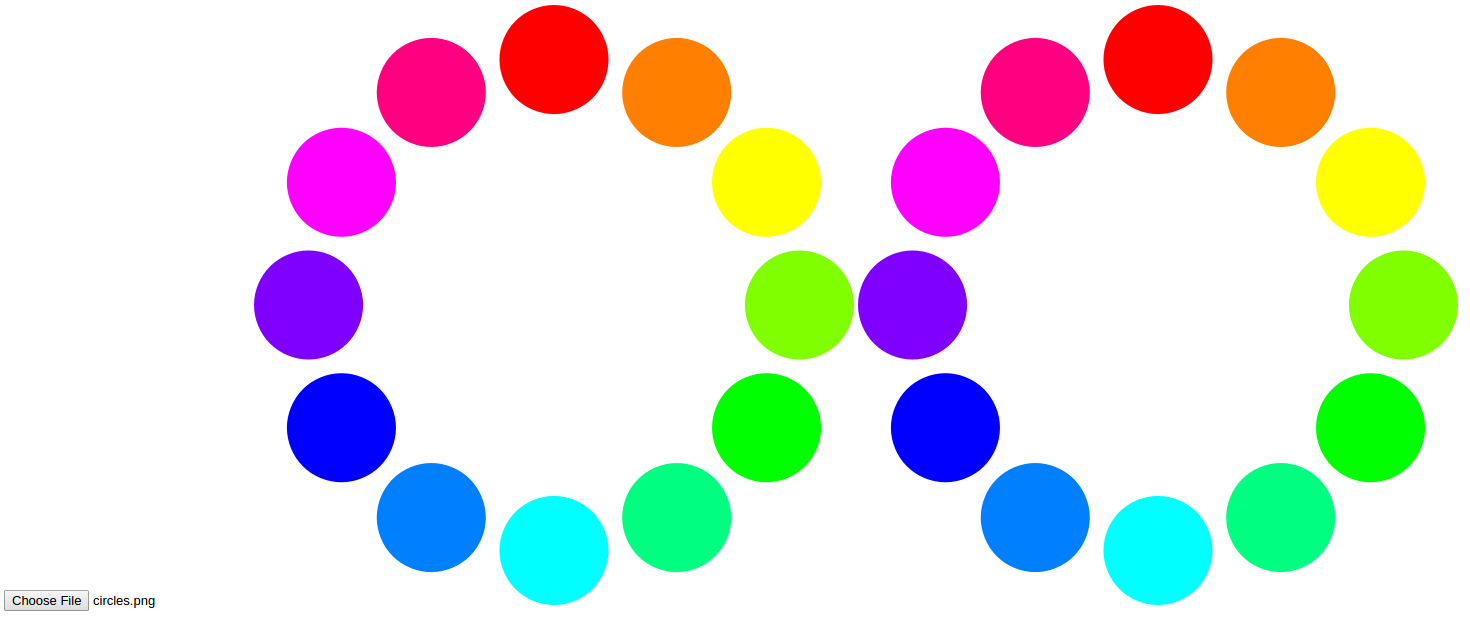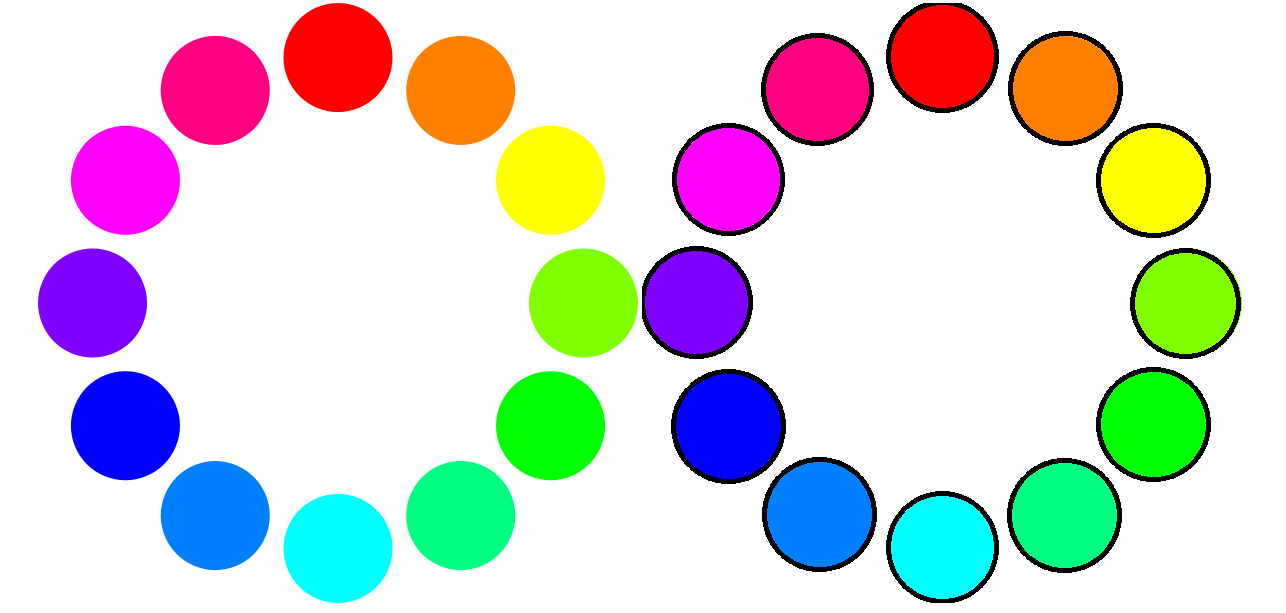# An Introduction to Computer Vision in JavaScript using OpenCV.js

Published on December 12, 2019 · Updated on October 20, 2020By Lily Rae### Introduction

OpenCV, or Open Source Computer Vision Library, is a powerful library used for image processing and image recognition. The library has a massive community and has been used extensively in many fields, from face detection to interactive art. It was first built in C++, but bindings have been created for different languages, such as Python and Java. It is even available in JavaScript as OpenCV.js, which is what we’ll be using for this tutorial.

In this project, we will create a webpage where a user can upload an image in order to detect all the circles contained in it. We will highlight the circles with a black outline, and the user will be able to download the modified image.

The code for this project is available in this GitHub repo.

## Prerequisites

To complete this tutorial, you will need to pull in the OpenCV.js library. The 3.3.1 version is available here:

``````https://docs.opencv.org/3.3.1/opencv.js
``````

Save this file locally as `opencv.js` in a place where you can easily locate it.

## Step 1 — Setting Up the Project

To get started, you will first need to create a space for your project. Create a directory named `opencvjs-project`:

``````mkdir opencvjs-project
``````

Move your local copy of `opencv.js` to this directory.

Next, add an `index.html` file with the following template:

index.html
``````<!DOCTYPE html>
<html>
<title>OpenCV.js</title>
<body>

<!-- Our HTML will go here-->

<script type="text/javascript">
// Our JavaScript code will go here
</script>

</body>
</html>
``````

In addition to the existing empty `<script>` tag in this file, add a new `<script>` tag which references the local `opencv.js` file. The script is quite large and takes a bit of time to load, so it is better load it asynchronously. This can be done by adding `async` to the `<script>` tag:

index.html
``````  <script type="text/javascript">
// Our JavaScript code will go here
</script>
<script async src="opencv.js" type="text/javascript"></script>
``````

As OpenCV.js may not be ready immediately due to the file size, we can provide a better user experience by showing that the content is being loaded. We can add a loading spinner to the page (credit to Sampson on StackOverflow).

First, add a `<div>` element `<body>`:

index.html
``````<body>

<!-- Our HTML will go here-->
<div class="modal"></div>

<script type="text/javascript">
// Our JavaScript code will go here
</script>
<script async src="opencv.js" type="text/javascript"></script>

</body>
``````

Next, add the following CSS into a separate `<style>` tag in the `<head>` of `index.html`. The spinner is invisible by default (thanks to `display: none;`):

index.html
``````/* display loading gif and hide webpage */
.modal {
display:    none;
position:   fixed;
z-index:    1000;
top:        0;
left:       0;
height:     100%;
width:      100%;
background: rgba( 255, 255, 255, .8)
url('http://i.stack.imgur.com/FhHRx.gif')
50% 50%
no-repeat;
}

/* prevent scrollbar from display during load */
overflow: hidden;
}

display: block;
}
``````

To show the loading gif, we can add the `"loading"` class to the body. Add the following to the empty `<script>`.

index.html
``````document.body.classList.add('loading');
``````

When OpenCV.js loads, we’ll want to hide the loading gif. Modify the `<script>` tag which references the local `opencv.js` file to add an `onload` event listener:

index.html
``````<script async src="opencv.js" onload="onOpenCvReady();" type="text/javascript"></script>
``````

Then add `onOpenCvReady` to the other `<script>` tag to handle removing the `"loading"` class:

index.html
``````// previous code is here

}
``````

Open the HTML page in your browser and check that OpenCV.js loads as expected.

Note: Using your browser’s developer tools, you should verify that there are no error messages in the Console tab and that the Network tab shows the `opencv.js` file being referenced properly. You will be periodically refreshing this page in your browser to view your latest changes.

Now that you’ve set up the project, you’re ready to build the image upload functionality.

To create the upload functionality, start by adding in an `<input>` element to `index.html`:

index.html
``````<input type="file" id="fileInput" name="file" />
``````

If we just want to display the source image, we’ll also need to add an `<img>` element and an event listener, which responds to change on the `<input>` element. Copy the following tag and place it under the `<input>` tag:

index.html
``````<img id="imageSrc" alt="No Image" />
``````

Get both the `<img>` element and the `<input>` element using their `id` values:

index.html
``````// previous code is here

let imgElement = document.getElementById('imageSrc');
let inputElement = document.getElementById('fileInput');
``````

Now, add the event listener, which triggers when the `<input>` changes (i.e., when a file is uploaded). From the change event, it’s possible to access the uploaded file (`event.target.files`), and convert it into a URL using `URL.createObjectURL`). The image’s `src` attribute can be updated to this URL:

index.html
``````// previous code is here

inputElement.onchange = function() {
imgElement.src = URL.createObjectURL(event.target.files);
};
``````Next to the original image, we can display a second image indicating the detected circles. The image will be displayed with a `<canvas>` element, which is used for drawing graphics with JavaScript:

index.html
``````<canvas id="imageCanvas"></canvas>
``````

We can add another event listener which updates the `<canvas>` with the uploaded image:

index.html
``````// previous code is here

cv.imshow('imageCanvas', image);
image.delete();
};
``````In this step, you’ve set up the image upload and display functionality. In the next step, you’ll explore how to use OpenCV to detect circles.

## Step 3 — Detecting Circles

This is where the power of OpenCV is evident, as detecting circles is a built-in task. We want to find the circles when the user clicks a button, so we’ll need to add the button and an event listener:

index.html
``````<button type="button" id="circlesButton" class="btn btn-primary">Circle Detection</button>
``````
index.html
``````// previous code is here

document.getElementById('circlesButton').onclick = function() {
// circle detection code
};
``````

Depending on the image, circle detection may take a while, so it is a good idea to disable the button to prevent the user from hitting it multiple times. It could also be useful to show a loading spinner on the button. We can reuse the loading gif from the initial script load:

index.html
``````// previous code is here

document.getElementById('circlesButton').onclick = function() {
this.disabled = true;

// circle detection code

this.disabled = false;
};
``````

The first step to detecting the circles is reading the image from the `<canvas>`.

In OpenCV, images are stored and manipulated as `Mat` objects. These are essentially matrices that hold values for each pixel in the image.

For our circle detection, we’re going to need three Mat objects:

• `srcMat` - Holds the source image (from which circles are detected)
• `circlesMat` - Stores the circles we detect
• `displayMatOne` - Displays to the user (on which we will draw our highlighted circles)

For the final `Mat`, we can make a copy of the first using the `clone` function:

index.html
``````// circle detection code
let displayMat = srcMat.clone();
let circlesMat = new cv.Mat();
``````

The `srcMat` needs to be converted to grayscale. This makes circle detection faster by simplifying the image. We can use `cvtColor` function to do this.

This function needs:

• the source `Mat` (`srcMat`)
• the destination `Mat` (in this case, the source and the destination Mat will be the same `srcMat`)
• a value which refers to the color conversion. `cv.COLOR_RGBA2GRAY` is the constant for grayscale.
index.html
``````cv.cvtColor(srcMat, srcMat, cv.COLOR_RGBA2GRAY);
``````

The `cvtColor` function, like other OpenCV.js functions, accepts more parameters. These are not required, so they will be set to the default. You can refer to the documentation for better customization.

Once the image is converted to grayscale, it’s possible to use the `HoughCircles` function to detect the circles.

This function needs:

• a source `Mat` from where it’ll find the circles (`srcMat`)
• a destination `Mat` where it’ll store the circles (`circlesMat`)
• the method to detect circles (`cv.HOUGH_GRADIENT`)
• the inverse ratio of the accumulator resolution (`1`)
• the minimum distance between the center point of circles (`45`)

There are more parameters, thresholds for the algorithm (`75` and `40`), which can be played with to improve accuracy for your images.

It is also possible to limit the range of the circles you want to detect by setting a minimum (`0`) and maximum radius (`0`).

index.html
``````cv.HoughCircles(srcMat, circlesMat, cv.HOUGH_GRADIENT, 1, 45, 75, 40, 0, 0);
``````

Now, we should have a `Mat` object with the circles detected in it.

Next, we’ll draw the circles in our `<canvas>`.

## Step 4 — Drawing Circles

All the circles which were detected can now be highlighted. We want to make an outline around each circle to show to the user. To draw a circle with OpenCV.js, we need the center point and the radius. These values are stored inside `circlesMat`, so we can retrieve it by looping through the matrix’s columns:

index.html
``````for (let i = 0; i < circlesMat.cols; ++i) {
// draw circles
}
``````

The `circlesMat` stores the `x` and `y` values for the center point and the radius sequentially.

For example, for the first circle, it would be possible to retrieve the values as follows:

``````let x = circlesMat.data32F;
let y = circlesMat.data32F;
``````

To get all the values for each circle, we can do the following:

index.html
``````for (let i = 0; i < circlesMat.cols; ++i) {
let x = circlesMat.data32F[i * 3];
let y = circlesMat.data32F[i * 3 + 1];
let radius = circlesMat.data32F[i * 3 + 2];

// draw circles
}
``````

Finally, with all these values, we are able to draw outlines around the circles.

To draw circles in OpenCV.js, we need:

• a destination `Mat` (the image we’re going to display to the user - `displayMat`)
• a center `Point` (using the x and y values)
• a scalar (an array of RGB values)

There are also additional parameters which can be passed into `circles`, such as the line thickness, which for in this example is `3`:

index.html
``````let center = new cv.Point(x, y);
cv.circle(displayMat, center, radius, [0, 0, 0, 255], 3);
``````

All the code for drawing circles is as follows:

index.html
``````for (let i = 0; i < circlesMat.cols; ++i) {
let x = circlesMat.data32F[i * 3];
let y = circlesMat.data32F[i * 3 + 1];
let radius = circlesMat.data32F[i * 3 + 2];
let center = new cv.Point(x, y);

// draw circles
cv.circle(displayMat, center, radius, [0, 0, 0, 255], 3);
}
``````

Once we’re done drawing all the circles on `displayMat`, we can display it to the user:

index.html
``````cv.imshow('imageCanvas', displayMat);
``````Finally, it’s good practice to clean up the `Mat` objects which we’ll no longer be needing. This is done to prevent memory problems:

index.html
``````srcMat.delete();
displayMat.delete();
circlesMat.delete();
``````

When we put it all together, the circle detection and drawing code will look like this:

index.html
``````// previous code is here

document.getElementById('circlesButton').onclick = function() {
this.disabled = true;

let displayMat = srcMat.clone();
let circlesMat = new cv.Mat();

cv.cvtColor(srcMat, srcMat, cv.COLOR_RGBA2GRAY);

cv.HoughCircles(srcMat, circlesMat, cv.HOUGH_GRADIENT, 1, 45, 75, 40, 0, 0);

for (let i = 0; i < circlesMat.cols; ++i) {
let x = circlesMat.data32F[i * 3];
let y = circlesMat.data32F[i * 3 + 1];
let radius = circlesMat.data32F[i * 3 + 2];
let center = new cv.Point(x, y);

// draw circles
cv.circle(displayMat, center, radius, [0, 0, 0, 255], 3);
}

cv.imshow('imageCanvas', displayMat);

srcMat.delete();
displayMat.delete();
circlesMat.delete();

this.disabled = false;
};
``````

With that, you have added the logic to detect and draw circles around circles in images.

After the image has been modified, the user may want to download it. To do this, add a hyperlink to your `index.html` file:

index.html
``````<a href="#" id="downloadButton">Download Image</a>
``````

We set the `href` to the image URL and the `download` attribute to the image filename. Setting the `download` attribute indicates to the browser that the resource should be downloaded rather than navigating to it. We can create the image URL from the `<canvas>` using the function `toDataURL()`.

Add the following JavaScript to the bottom of the `<script>`:

index.html
``````// previous code is here

this.href = document.getElementById('imageCanvas').toDataURL();
};
``````

## Conclusion

Detecting circles is possible with OpenCV. Once you get accustomed to manipulating images as `Mat` objects, there is so much more you can do. The `HoughCircles` algorithm is one of many provided by OpenCV to make image processing and image recognition that much easier.

You can find more tutorials, including face recognition and template matching, on the OpenCV website. You can also read more about computer vision by visiting the machine learning topic page.

Thanks for learning with the DigitalOcean Community. Check out our offerings for compute, storage, networking, and managed databases.Lily Rae

author#### Still looking for an answer?

Ask a questionSearch for more help

﻿

You can type !ref in this text area to quickly search our full set of tutorials, documentation & marketplace offerings and insert the link!

Extraordinary approximation to use open cv and javascript. Have you worked with react?. It would be awesome.